## Bandwidth of RLC Circuit:

The bandwidth of any system is the range of frequencies for which the current or output voltage is equal to 70.7% of its value at the resonant frequency, and it is denoted by BW. Figure 8.9 shows the response of a series Bandwidth of RLC Circuit.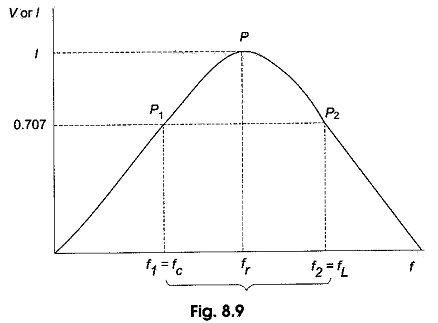Here the frequency f1 is the frequency at which the current is 0.707 times the current at resonant value, and it is called the lower cut-off frequency. The frequency f2 is the frequency at which the current is 0.707 times the current at resonant value (i.e. maximum value), and is called the upper cut-off frequency. The bandwidth, or BW, is defined as the frequency difference between f2 and f1.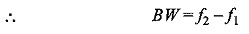The unit of BW is hertz (Hz).

If the current at P1 is 0.707Imax, the impedance of the Bandwidth of RLC Circuit at this point is √2 R, and henceSimilarly,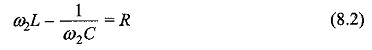If we equate both the above equations, we get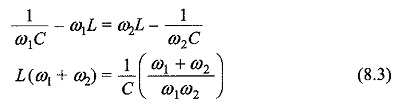From Eq. 8.3, we get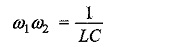we have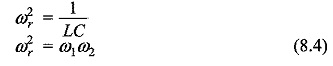If we add Eqs 8.1 and 8.2, we get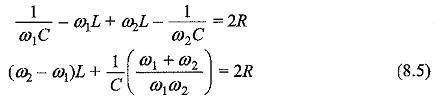Since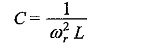and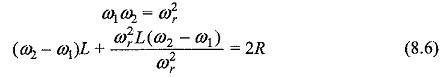From Eq. 8.6, we have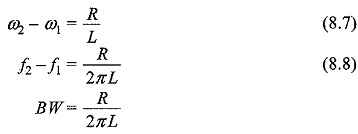or

From Eq. 8.9, we have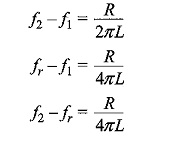The lower frequency limit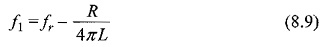The upper frequency limit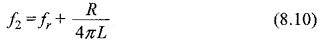If we divide the equation on both sides by fr, we get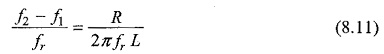Here an important property of a coil is defined. It is the ratio of the reactance of the coil to its resistance. This ratio is defined as the Q of the coil. Q is known as a figure of merit, it is also called quality factor and is an indication of the quality of a coil.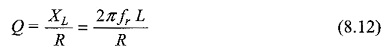If we substitute Eq. (8.11) in Eq. (8.12), we get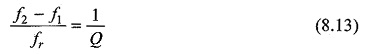The upper and lower cut-off frequencies are sometimes called the half-power frequencies. At these frequencies the power from the source is half of the power delivered at the resonant frequency.

At resonant frequency, the power is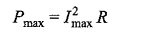At frequency f1, the power is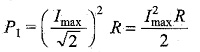Similarly, at frequency f2, the power is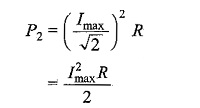The response curve in Fig. 8.9 is also called the selectivity curve of the Bandwidth of RLC Circuit. Selectivity indicates how well a resonant circuit responds to a certain frequency and eliminates all other frequencies. The narrower the bandwidth, the greater the selectivity.

Scroll to Top